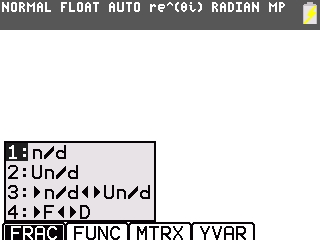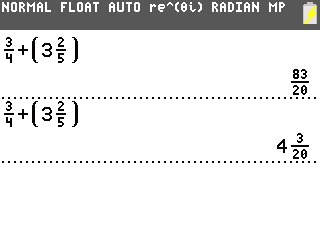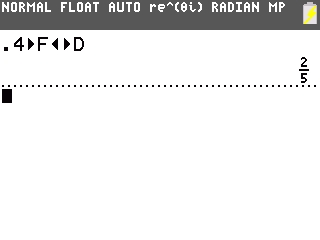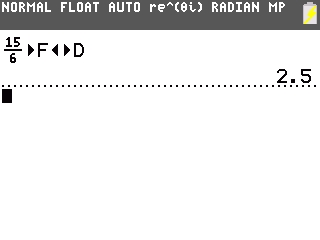# Knowledge Base

## Solution 34831: Entering and Computing Fractions on the TI-84 Plus Family of Graphing Calculators.

### How do I enter and compute fractions on the TI-84 Plus family of graphing calculators?

The TI-84 Plus family of graphing calculators do not have a specific fraction key, but does have a template that allows fractions to be entered.

For example, add 3 2/5 to 3/4 using the steps below:

1) Press [ALPHA] [F1] .2) Enter in .
3) Press [Down Arrow].
4) Enter in .
5) Press [Right Arrow].
6) Press [+].
7) Press [ALPHA] [F1] .
8) Enter in .
9) Press [Right Arrow].
10) Enter in .
11) Press [Down Arrow].
12) Enter in .
13) Press [ENTER].

Two possible answers can be displayed with MATHPRINT turned on and switching from n/d to Un/d in the [Mode] settings.Changing a decimal into a fraction.

Example: Convert .4 into a fraction.

Press [.]  [ALPHA] [F1]  [Enter].Changing a fraction into a decimal.

Example: Convert 15/6 into a decimal.

1) Press [ALPHA] [F1] .
2) Enter in .
3) Press [Down Arrow].
4) Enter in .
5) Press [Right Arrow].
6) Press [ALPHA] [F1]  [Enter].